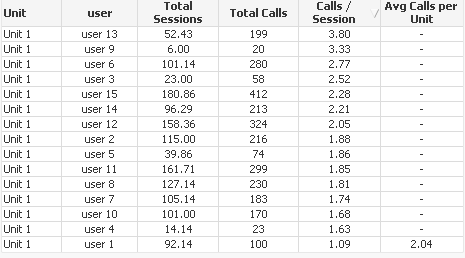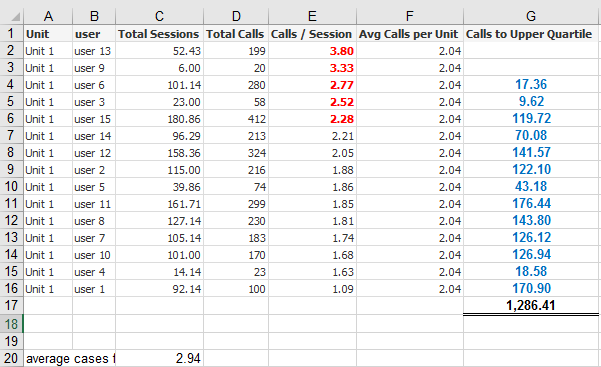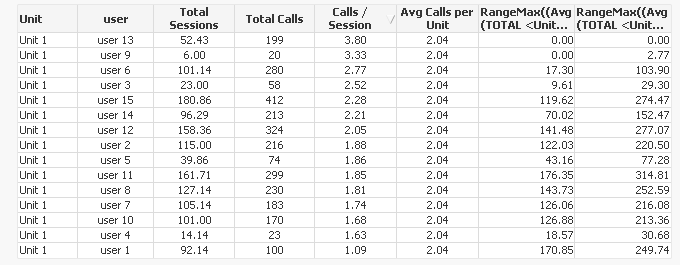# QlikView App Development

Discussion Board for collaboration related to QlikView App Development.

Announcements
Coming your way, the Qlik Data Revolution Virtual Summit. October 27-29. REGISTER
cancel
Showing results for
Did you mean:
HighlightedNot applicable

## Aggregate & Upper Quartile Help

Hi,

In the attached sample app I have the following straight table :I 'think' I have calculated the 'AVG Calls per Unit' correctly by using an aggregate function. Is there any reason why it is only showing in one cell?

Also,  I need to create another expression where I need to calculate if each User carried out at least the average number of Calls for the unit - how many more calls could be made for this particular Unit.

Following on from this.  If each user carried out the total calls per session at least equal to the upper quartile (top 5) of users how many more calls could be made ?

Any help or advice on this is greatly appreciated.

Many thanks!

1 Solution

Accepted Solutions
HighlightedMVP

Try this:

RangeMax((Avg(TOTAL <Unit> Aggr(If(Rank(total_calls/total_sessions) < 6, total_calls/total_sessions), Unit, user)) - total_calls/total_sessions) * total_sessions, 0)

31 Replies
HighlightedMVP

=Sum(TOTAL <Unit> total_calls)/Sum(TOTAL <Unit> total_sessions)

HighlightedNot applicable

Thanks for your quick reply!  Any ideas on the upper quartile part of my query?

HighlightedMVP

What is the expected output you are hoping to see and where?

HighlightedNot applicable

Hi,

In the example below, I have taken the average of the Units's top 5 user's 'calls per session' which equals '2.94', then I need to create a new expression 'Calls to Upper Quartile' whereby for every user below 2.94 it calculates how many more calls could be made if they matched the rate of the top 5 :

if(user rate < average of top 5 , ([top 5 average] - [Calls/Session]) *  Total Sessions)

This will be need to be calculated per unit...many thanks for any help again - its really appreciated.

HighlightedMVP

Try this:

RangeMax((Avg(TOTAL <Unit> Aggr(If(Rank(total_calls/total_sessions) < 6, total_calls/total_sessions), Unit, user)) - total_calls/total_sessions) * total_sessions, 0)

HighlightedNot applicable

Perfect ! that's brilliant!!

One last thing - could a final expression be created to find the maximum calls/per session for that unit and create another column similar to the top 5?

Thanks again - your help has been appreciated.!

HighlightedMVP

May be this?

RangeMax((Avg(TOTAL <Unit> Aggr(If(Rank(total_calls/total_sessions) < 2, total_calls/total_sessions), Unit, user)) - total_calls/total_sessions) * total_sessions, 0)HighlightedNot applicable

Will this solution also work in a pivot table with set analaysis within the expressions?  I have tried to apply your solutions to my actual document and not getting the correct results....

Dimensions :  Unit

User

My actual current expressions :

Total Sessions : =num(sum({< [DateValue]={'>=\$(=date(vStart))<=\$(=date(vEnd))'}  >}benchmark_total_sessions),'0')

Total Calls : =sum({< [DateValue]={'>=\$(=date(vStart))<=\$(=date(vEnd))'} >}benchmark_calls_undertaken)

Calls/Session :  =[Total Calls]/[Total Sessions(which uses the two expressions)

Any ideas?

Many thanks again for your time

HighlightedMVP

I believe this should work...

RangeMax((Avg(TOTAL <Unit> Aggr(If(Rank(sum({< [DateValue]={'>=\$(=date(vStart))<=\$(=date(vEnd))'} >}benchmark_calls_undertaken)/sum({< [DateValue]={'>=\$(=date(vStart))<=\$(=date(vEnd))'}  >}benchmark_total_sessions)) < 2, sum({< [DateValue]={'>=\$(=date(vStart))<=\$(=date(vEnd))'} >}benchmark_calls_undertaken)/sum({< [DateValue]={'>=\$(=date(vStart))<=\$(=date(vEnd))'}  >}benchmark_total_sessions)), Unit, user)) - sum({< [DateValue]={'>=\$(=date(vStart))<=\$(=date(vEnd))'} >}benchmark_calls_undertaken)/sum({< [DateValue]={'>=\$(=date(vStart))<=\$(=date(vEnd))'}  >}benchmark_total_sessions)) * sum({< [DateValue]={'>=\$(=date(vStart))<=\$(=date(vEnd))'}  >}benchmark_total_sessions), 0)

But I would let you do the testing. In case it doesn't work, please provide an update sample for us to look at.

Best,

Sunny